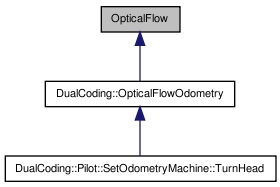Tekkotsu Homepage Demos Overview Downloads Dev. Resources Reference Credits

# OpticalFlow Class Reference

`#include <OpticalFlow.h>`

Inheritance diagram for OpticalFlow:[legend]

## Detailed Description

Definition at line 30 of file OpticalFlow.h.

List of all members.

## Public Member Functions

OpticalFlow ()
OpticalFlow (OpticalFlow &other)
virtual ~OpticalFlow ()
void updateFlow ()
Update the current flow vectors and increment the integrated angle.
void drawFlow ()
Draw the optical flow vectors in the camera shape space.
void swapPyramids ()
void initializePositions ()
Select the features that we are going to track.
float getIntegratedFlow ()
void computeRelevanceScores ()
Compute scores for vectors to determine outliers.

## Static Public Member Functions

static bool myFlowComp (const FlowVector &v1, const FlowVector &v2)
Comparison function to sort the flow vectors by horizontal translation.
static bool myFlowComp2 (const FlowVector &v1, const FlowVector &v2)
Comparison function in order to sort the flow vectors by relevance score.
static bool scoreComp (const ScorePoint &p1, const ScorePoint &p2)
static fmat::Column< 2 > iterativeLucasKanade (fmat::Column< 2 > center, fmat::Column< 2 > trans, RawImage &img1, RawImage &img2, int window)
Lucas-Kanade optic flow algorithm.

## Static Public Attributes

static const unsigned int SCORE_LAYER = 0
Image layer from which we select features to track.
static const unsigned int NUM_FLOW_VECTORS = 50
Number of flow vectors to calculate.
static const unsigned int FLOW_WINDOW = 2
Size of width/2, height/2 of the feature when we calculate flow.
static const unsigned int SCORE_WINDOW = 1
Size of width/2, height/2 of the feature when we select them for flow calculation.
static const unsigned int NUM_FRAMES = 10
Number of frames we remember about the history of the robot's motion. Used for removing outliers.
static const unsigned int CANDIDATE_FEATURE_RESOLUTION = 30
Number of pixels per grid square when calculating minimum eigenvalues.

## Protected Attributes

float integratedFlow

## Private Member Functions

OpticalFlowoperator= (const OpticalFlow &other)

## Private Attributes

std::vector< FlowVectorflowVectors
std::vector< DualCoding::Shape
< DualCoding::LineData > >
flowVectorVisuals
std::vector< floatpastTranslations
RawImagePyramid pyramid1
RawImagePyramid pyramid2
RawImagePyramidcurrPyramid
RawImagePyramidprevPyramid

## Constructor & Destructor Documentation

 OpticalFlow::OpticalFlow ( )

Definition at line 6 of file OpticalFlow.cc.

 OpticalFlow::OpticalFlow ( OpticalFlow & other )
 virtual OpticalFlow::~OpticalFlow ( ) ` [virtual]`

Definition at line 57 of file OpticalFlow.h.

## Member Function Documentation

 void OpticalFlow::computeRelevanceScores ( )

Compute scores for vectors to determine outliers.

The lower the score, the better. Uses the idea that the robot's motion is continuous. Gives high score to vectors that are far away from the robot's history of motion.

Definition at line 19 of file OpticalFlow.cc.

Referenced by updateFlow().

 void OpticalFlow::drawFlow ( )

Draw the optical flow vectors in the camera shape space.

Definition at line 76 of file OpticalFlow.cc.

 float OpticalFlow::getIntegratedFlow ( )

Definition at line 83 of file OpticalFlow.h.

 void OpticalFlow::initializePositions ( )

Select the features that we are going to track.

The features are scored by the magnitude of their minimum eigenvalue. Then, the features wpith the largest score are selected.

Definition at line 97 of file OpticalFlow.cc.

Referenced by updateFlow().

 fmat::Column< 2 > OpticalFlow::iterativeLucasKanade ( fmat::Column< 2 > center, fmat::Column< 2 > trans, RawImage & img1, RawImage & img2, int window ) ` [static]`

Lucas-Kanade optic flow algorithm.

Definition at line 131 of file OpticalFlow.cc.

Referenced by updateFlow().

 bool OpticalFlow::myFlowComp ( const FlowVector & v1, const FlowVector & v2 ) ` [static]`

Comparison function to sort the flow vectors by horizontal translation.

Definition at line 10 of file OpticalFlow.cc.

Referenced by updateFlow().

 bool OpticalFlow::myFlowComp2 ( const FlowVector & v1, const FlowVector & v2 ) ` [static]`

Comparison function in order to sort the flow vectors by relevance score.

Definition at line 14 of file OpticalFlow.cc.

Referenced by updateFlow().

 OpticalFlow& OpticalFlow::operator= ( const OpticalFlow & other ) ` [private]`
 static bool OpticalFlow::scoreComp ( const ScorePoint & p1, const ScorePoint & p2 ) ` [static]`

Definition at line 65 of file OpticalFlow.h.

Referenced by initializePositions().

 void OpticalFlow::swapPyramids ( )

Definition at line 65 of file OpticalFlow.cc.

Referenced by updateFlow().

 void OpticalFlow::updateFlow ( )

Update the current flow vectors and increment the integrated angle.

Definition at line 29 of file OpticalFlow.cc.

Referenced by DualCoding::OpticalFlowOdometry::update().

## Member Data Documentation

 const unsigned int OpticalFlow::CANDIDATE_FEATURE_RESOLUTION = 30` [static]`

Number of pixels per grid square when calculating minimum eigenvalues.

Definition at line 52 of file OpticalFlow.h.

Referenced by initializePositions().

 RawImagePyramid* OpticalFlow::currPyramid` [private]`

Definition at line 108 of file OpticalFlow.h.

Referenced by swapPyramids(), and updateFlow().

 const unsigned int OpticalFlow::FLOW_WINDOW = 2` [static]`

Size of width/2, height/2 of the feature when we calculate flow.

The larger this is, the better the tracking. Large values will severely decrease framerate, however.

Definition at line 41 of file OpticalFlow.h.

Referenced by updateFlow().

 std::vector OpticalFlow::flowVectors` [private]`

Definition at line 100 of file OpticalFlow.h.

Referenced by computeRelevanceScores(), drawFlow(), initializePositions(), and updateFlow().

 std::vector > OpticalFlow::flowVectorVisuals` [private]`

Definition at line 101 of file OpticalFlow.h.

Referenced by drawFlow().

 float OpticalFlow::integratedFlow` [protected]`

Definition at line 97 of file OpticalFlow.h.

 const unsigned int OpticalFlow::NUM_FLOW_VECTORS = 50` [static]`

Number of flow vectors to calculate.

Definition at line 36 of file OpticalFlow.h.

Referenced by computeRelevanceScores(), initializePositions(), and updateFlow().

 const unsigned int OpticalFlow::NUM_FRAMES = 10` [static]`

Number of frames we remember about the history of the robot's motion. Used for removing outliers.

Definition at line 49 of file OpticalFlow.h.

Referenced by computeRelevanceScores(), and updateFlow().

 std::vector OpticalFlow::pastTranslations` [private]`

Definition at line 102 of file OpticalFlow.h.

Referenced by computeRelevanceScores(), and updateFlow().

 RawImagePyramid* OpticalFlow::prevPyramid` [private]`

Definition at line 109 of file OpticalFlow.h.

Referenced by swapPyramids(), and updateFlow().

 RawImagePyramid OpticalFlow::pyramid1` [private]`

Definition at line 106 of file OpticalFlow.h.

Referenced by swapPyramids().

 RawImagePyramid OpticalFlow::pyramid2` [private]`

Definition at line 107 of file OpticalFlow.h.

Referenced by swapPyramids().

 const unsigned int OpticalFlow::SCORE_LAYER = 0` [static]`

Image layer from which we select features to track.

Definition at line 34 of file OpticalFlow.h.

Referenced by initializePositions().

 const unsigned int OpticalFlow::SCORE_WINDOW = 1` [static]`

Size of width/2, height/2 of the feature when we select them for flow calculation.

It is typically safe to keep this low to save computation time.

Definition at line 46 of file OpticalFlow.h.

Referenced by initializePositions().

The documentation for this class was generated from the following files:

 Tekkotsu v5.1CVS Generated Mon May 9 04:59:13 2016 by Doxygen 1.6.3